# Attribute charts for monitoring the mean vector of

• Slides: 26Attribute charts for monitoring the mean vector of bivariate processes Antonio F. B. Costa UNESP São Paulo State University Linda Lee Ho USP São Paulo UniversityUnivariate Case Go-No-Go Gauge Montgomery’s Book: X~N(m 0=50; s 0=2) m 1=52 (power=0. 50) LSL=44 USL=56 The chart requires samples of size 9 The np chart requires samples of size 60Hu, Z. and Jiao, J. (2008) A control chart for monitoring mean based on attibute inspection, IJPR. Discriminating Warning Limits The second-class unit is not necessarely non conforming The np chart might be designed to signal an out of control condition even before any defective is produced. (0)Shewhart model REYNOLDS JUNIOR, M. R. ; ARNOLD, J. C. ; BAIK, J. W. (1996) Variable sampling interval X charts n the presence of correlation. Journal of Quality Technology, v. 28, n. 1, p. 12 -29. Wandering process mean Walter Andrew Shewhart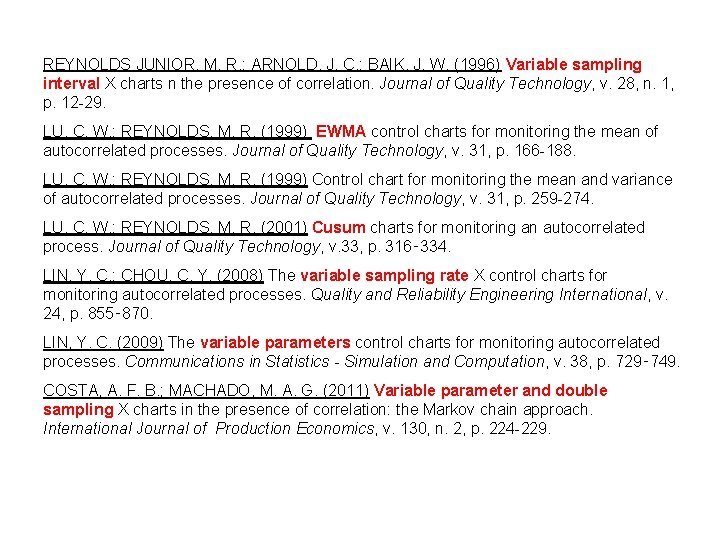REYNOLDS JUNIOR, M. R. ; ARNOLD, J. C. ; BAIK, J. W. (1996) Variable sampling interval X charts n the presence of correlation. Journal of Quality Technology, v. 28, n. 1, p. 12 -29. LU, C. W. ; REYNOLDS, M. R. (1999) EWMA control charts for monitoring the mean of autocorrelated processes. Journal of Quality Technology, v. 31, p. 166 -188. LU, C. W. ; REYNOLDS, M. R. (1999) Control chart for monitoring the mean and variance of autocorrelated processes. Journal of Quality Technology, v. 31, p. 259 -274. LU, C. W. ; REYNOLDS, M. R. (2001) Cusum charts for monitoring an autocorrelated process. Journal of Quality Technology, v. 33, p. 316‑ 334. LIN, Y. C. ; CHOU, C. Y. (2008) The variable sampling rate X control charts for monitoring autocorrelated processes. Quality and Reliability Engineering International, v. 24, p. 855‑ 870. LIN, Y. C. (2009) The variable parameters control charts for monitoring autocorrelated processes. Communications in Statistics - Simulation and Computation, v. 38, p. 729‑ 749. COSTA, A. F. B. ; MACHADO, M. A. G. (2011) Variable parameter and double sampling X charts in the presence of correlation: the Markov chain approach. International Journal of Production Economics, v. 130, n. 2, p. 224 -229.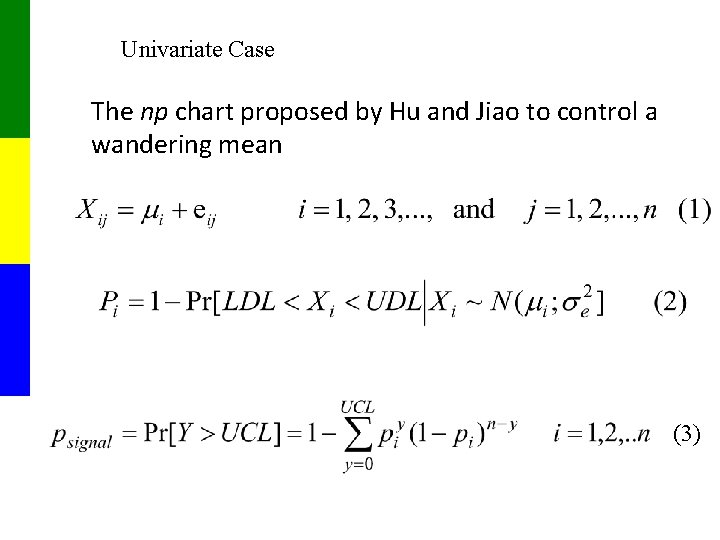Univariate Case The np chart proposed by Hu and Jiao to control a wandering mean (3)When the process mean wanders, a simple way to study the chart’s performance is building a Markov chain that allows us to express the ARL as a function of the expected number the transient states are visited. (6) (7)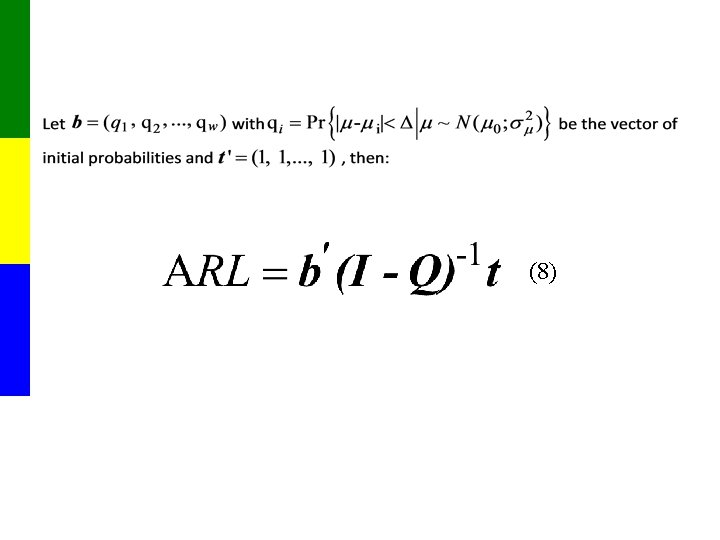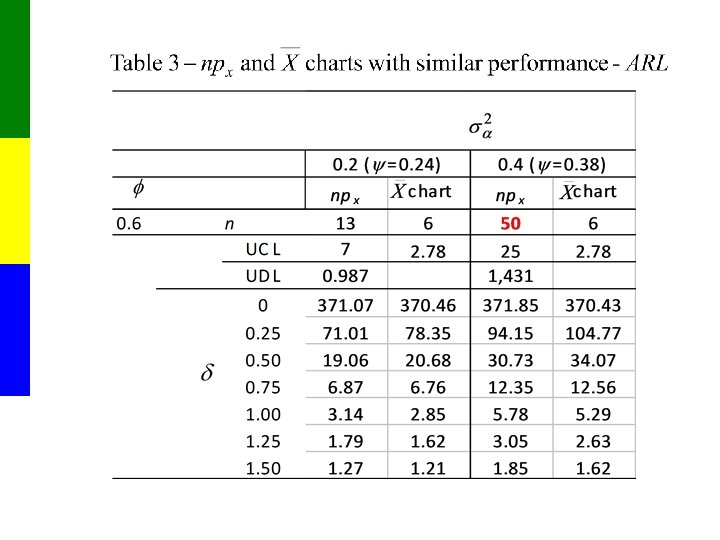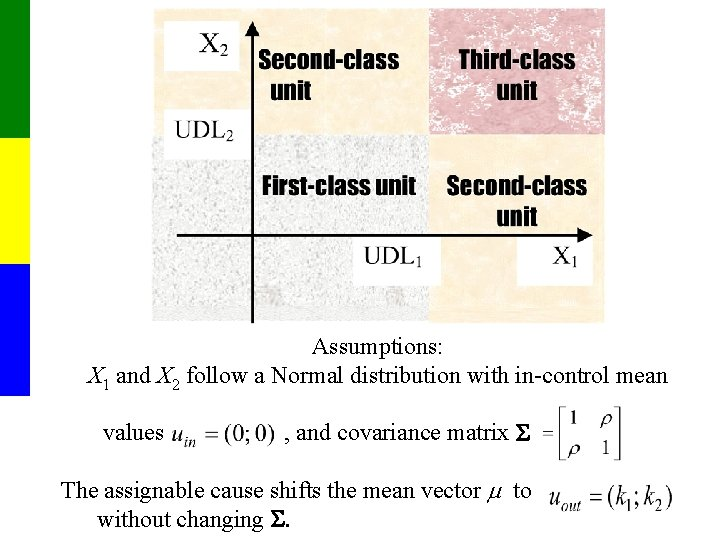Assumptions: X 1 and X 2 follow a Normal distribution with in-control mean values , and covariance matrix S The assignable cause shifts the mean vector m to without changing S.First proposed attribute chart is the npa chart, its monitoring statistic is A: the number of sample units with a second or a third-classification The npa chart with Upper Control Limit- UCL=m signals when A>m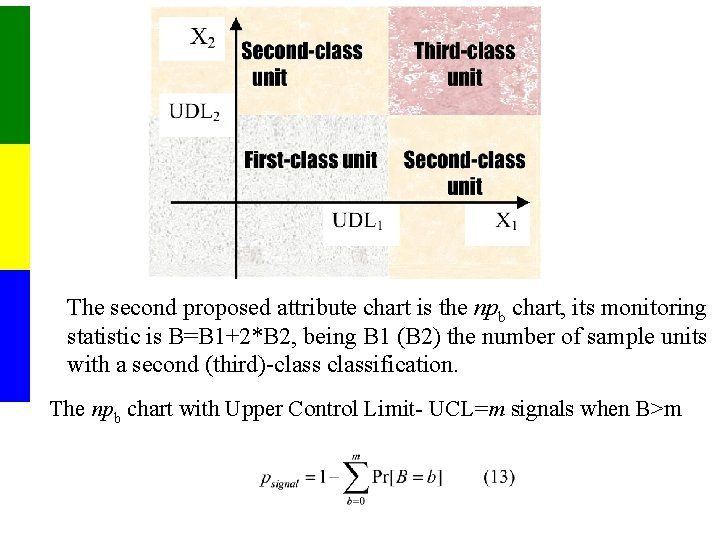The second proposed attribute chart is the npb chart, its monitoring statistic is B=B 1+2*B 2, being B 1 (B 2) the number of sample units with a second (third)-classification. The npb chart with Upper Control Limit- UCL=m signals when B>m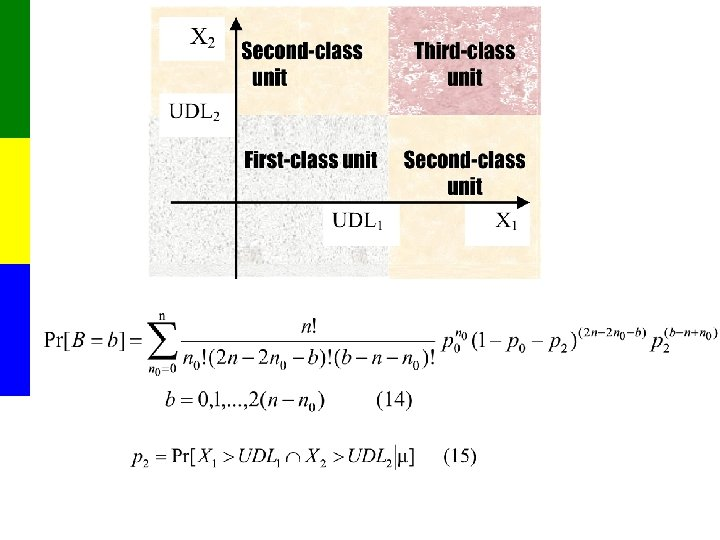Table 4: The ARL values (n=6 and =0)Table 5: The ARL values (n=6 and =0. 3)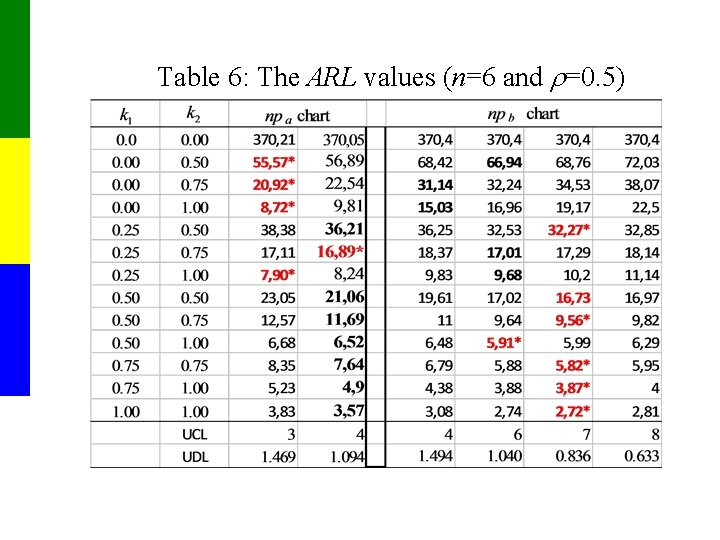Table 6: The ARL values (n=6 and =0. 5)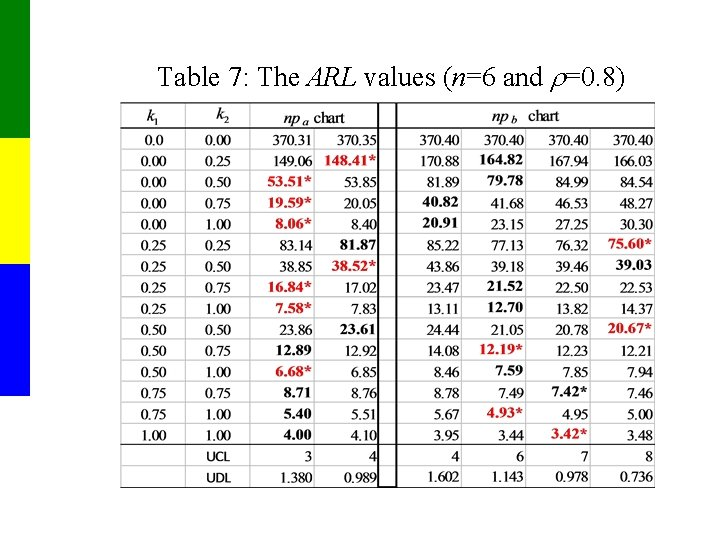Table 7: The ARL values (n=6 and =0. 8)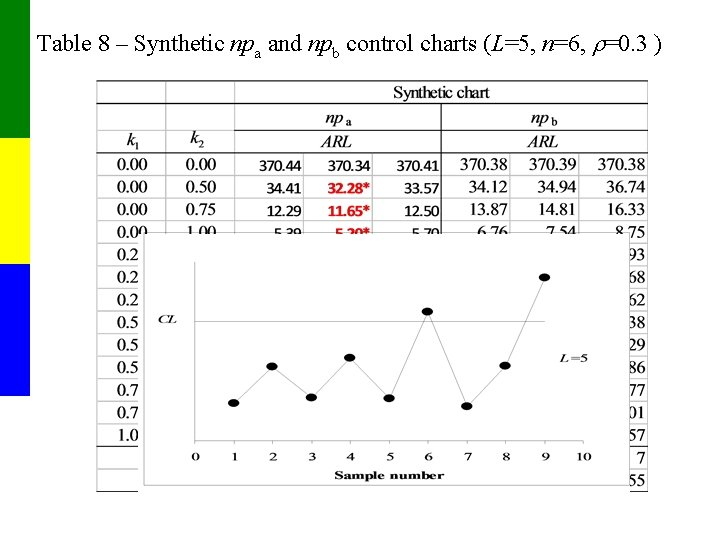Table 8 – Synthetic npa and npb control charts (L=5, n=6, =0. 3 )Table 9– Synthetic npa and npb control charts (L=5, n=6, =0. 8 )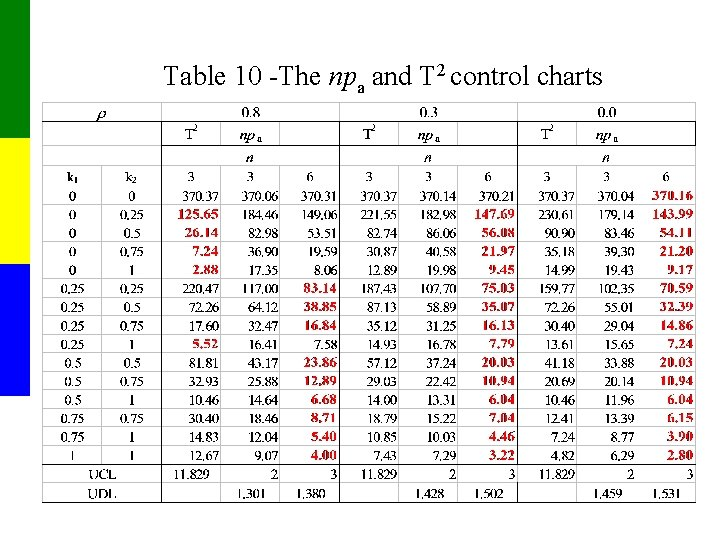Table 10 -The npa and T 2 control charts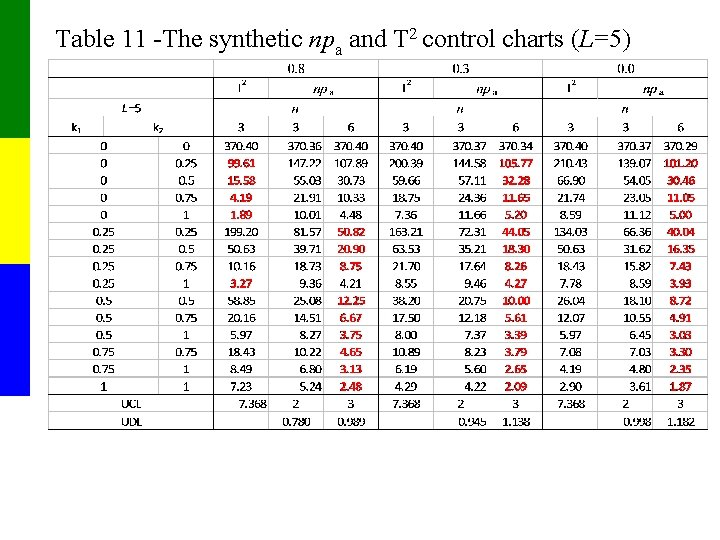Table 11 -The synthetic npa and T 2 control charts (L=5)Wandering mean vector